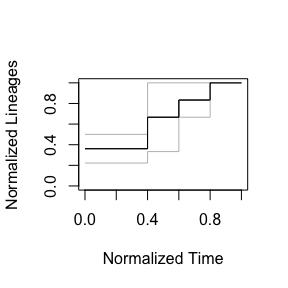# How to plot multiple nLTTs and their average

#### 2023-08-21

The nLTT package facilitates plotting one or more nLTT plots, using nllt_plot and nllt_lines.

These functions, however, do not show the average nLTT plot.

The function nltts_plot plots multiple nLTT plots and shows the average nLTT. This helps in getting a quick visualization of your data.

For working with the raw values, get_nltt_values is preferably used.

### Example: Easy trees

Create two easy trees:

newick1 <- "((A:1,B:1):2,C:3);"
newick2 <- "((A:2,B:2):1,C:3);"
phylogeny2 <- ape::read.tree(text = newick2)

There are very similar. phylogeny1 has short tips:

ape::plot.phylo(phylogeny1)
ape::add.scale.bar() #nolint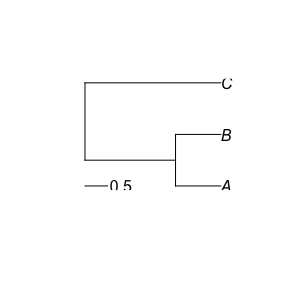This can be observed in the nLTT plot:

nLTT::nltt_plot(phylogeny1, ylim = c(0, 1))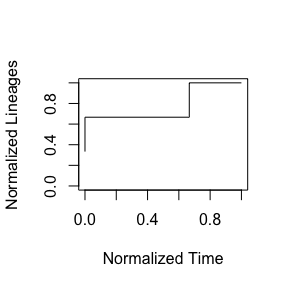phylogeny2 has longer tips:

ape::plot.phylo(phylogeny2)
ape::add.scale.bar() #nolint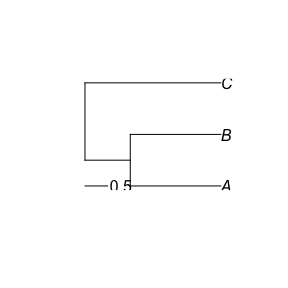Also this can be observed in the nLTT plot:

nLTT::nltt_plot(phylogeny2, ylim = c(0, 1))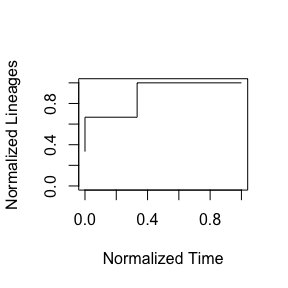The average nLTT plot should be somewhere in the middle.

The same, now shown as a plot:

nLTT::nltts_plot(c(phylogeny1, phylogeny2), dt = 0.20, plot_nltts = TRUE)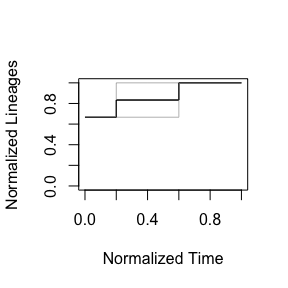### Example: Harder trees

Create two easy trees:

newick1 <- "((A:1,B:1):1,(C:1,D:1):1);"
newick2 <- paste0("((((XD:1,ZD:1):1,CE:2):1,(FE:2,EE:2):1):4,((AE:1,BE:1):1,",
"(WD:1,YD:1):1):5);"
)
phylogeny2 <- ape::read.tree(text = newick2)

There are different. phylogeny1 is relatively simple, with two branching events happening at the same time:

ape::plot.phylo(phylogeny1)
ape::add.scale.bar() #nolint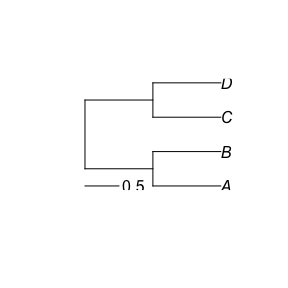This can be observed in the nLTT plot:

nLTT::nltt_plot(phylogeny1, ylim = c(0, 1))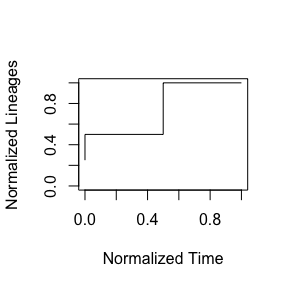phylogeny2 is more elaborate:

ape::plot.phylo(phylogeny2)
ape::add.scale.bar() #nolint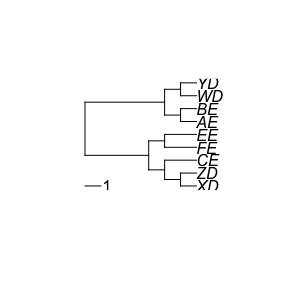Also this can be observed in the nLTT plot:

nLTT::nltt_plot(phylogeny2, ylim = c(0, 1))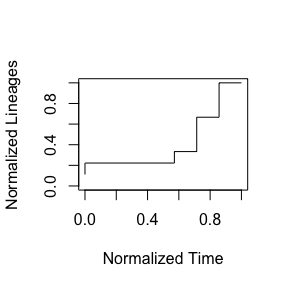The same, now shown as a plot:

nLTT::nltts_plot(
c(phylogeny1, phylogeny2),
dt = 0.20,
plot_nltts = TRUE
)